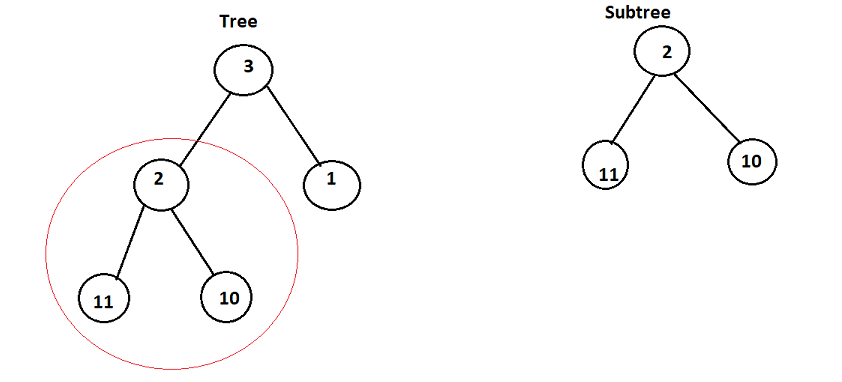# Subtree of Another Tree

Problem statement: Given the roots of two binary trees root and subRoot, return true if there is a subtree of root with the same structure and node values of subRoot and false otherwise.

Examples:

```In this example, you can see that we are having the subtree on the right inside the tree on the left. So the answer is true.```

### Solution:

Disclaimer: Don’t jump directly to the solution, try it out yourself first.

### Intuition:

In this problem, we can see that all we need to do is to locate the root of the subtree in the tree and then apply a traversal to check whether the two subtrees are equal or not. But that’s not always true. There is nowhere mentioned in the question that identical values cannot exist on the tree. So you need to check for every node whether the two subtrees match.

### Approach:

We need to check for every node whether the subtree beneath it matches the subtree given in the question. For this, we can use inorder traversal to generate all nodes of the given binary tree and then use another function to match the two subtrees. The matching function will be recursive and must have the following conditions:-

1. If any one of the nodes is null and the other one is not null then we return false.
2. If both the nodes are null then we need to return true.
3. If both the nodes are not null then in that case we need to check for the value of the given two nodes.

Code:

## C++ Code

``````#include <bits/stdc++.h>

using namespace std;

struct node {
int data;
struct node * left, * right;
};

struct node *newNode(int data) {
struct node *node = (struct node*) malloc(sizeof(struct node));
node -> data = data;
node -> left = NULL;
node -> right = NULL;

return (node);
}

bool ans = false;

bool match(node * root, node *subRoot) {
if (root && subRoot) {
bool a = match(root -> left, subRoot -> left);
bool b = match(root -> right, subRoot -> right);

if ((root -> data == subRoot -> data) && a && b)
return true;
else
return false;
} else if (!root && !subRoot) return true;
else return false;
}

void inorder(node * root, node * subRoot) {
if (root) {
inorder(root -> left, subRoot);
bool x = match(root, subRoot);
if (x) ans = 1;
inorder(root -> right, subRoot);
}
}

bool isSubtree(node * root, node * subRoot) {
inorder(root, subRoot);
return ans;
}

int main() {
struct node *root = newNode(3);
root->right = newNode(1);
root->left = newNode(2);
root->left->left = newNode(11);
root->left->right = newNode(10);

struct node *subRoot = newNode(2);
subRoot->left = newNode(11);
subRoot->right = newNode(10);

cout << boolalpha;
isSubtree(root, subRoot);
cout << ans << endl;
}
``````

Output: True

Time Complexity: O(N*K), where k = no. of nodes in subtree and n = no. of nodes in the tree

Space Complexity: O(1)